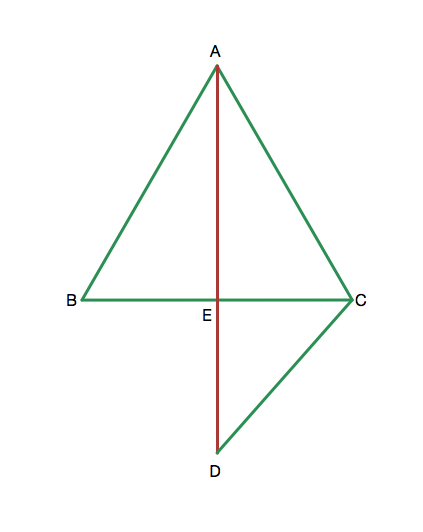# Equilateral Triangle Geometry

Geometry Level 1The equilateral triangle ABC has a height from A, which intersects BC at E. Extend AE to a point D such that AC=CD. Find the angles EBD and EDB in degrees.

×